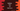# 3 ways in Python to calculate GCD or HCF of two numbers## How to calculate HCF or GCD of two numbers in Python:

HCF(Highest common factor) or GCD(greatest common divisor) is the largest positive integer which can divide each of the numbers when at least one of the numbers is not zero.

For example, let’s find the HCF of 9 and 18. The divisors of 9 are 1, 3, 9 and the divisors of 18 are 1, 3, 6, 9. So, the highest common factor or greatest common divisor of 9 and 18 is 9.

We will learn different ways to find the HCF of two numbers in Python in this example.

### Method 1: Python program to find the HCF of two numbers by using a for loop:

In this example, we will use a for loop that will run from 1 to the smaller number. On each iteration, it will check if the current value of the loop can divide both the numbers or not. If yes, it will assign that value to a variable that will hold the HCF value once the loop ends.

Let’s write down the program:

``````def get_hcf(first, second):
smaller_no = first

if second < first:
smaller_no = second

hcf = 1

for i in range(2, smaller_no + 1):
if first % i == 0 and second % i == 0:
hcf = i

return hcf

n1 = int(input("Enter the first number: "))
n2 = int(input("Enter the second number: "))

print(f'HCF of {n1} and {n2} is: {get_hcf(n1, n2)}')``````

Here,

• It takes the numbers as inputs from the user and assigns to the n1 and n2 variables.
• The get_hcf method is used to find the HCF of two numbers. It takes the numbers as its arguments and returns the HCF.
• It assigns the smaller number to the smaller_no variable. The for loop runs from 2 to smaller_no to find the HCF value. The HCF is assigned to the hcf variable.

If you run this program, it will print output as below:

``````Enter the first number: 13
Enter the second number: 52
HCF of 13 and 52 is: 13

Enter the first number: 12
Enter the second number: 46
HCF of 12 and 46 is: 2``````

### Method 2: Python program to find the HCF of two numbers by using a loop in reverse:

Instead of running a loop from 2 to the number, we can also run the loop in reverse, i.e. starting from the smaller number. Once we found any number, we can skip from the loop as that is the HCF.

This is a better approach than the previous one because it will reduce the number of loop steps.

Below is the complete program:

``````def get_hcf(first, second):
i = first

if second < first:
i = second

while (i > 0):
if first % i == 0 and second % i == 0:
return i
i = i - 1

n1 = int(input("Enter the first number: "))
n2 = int(input("Enter the second number: "))

print(f'HCF of {n1} and {n2} is: {get_hcf(n1, n2)}')``````

It will print similar output. In this program, i holds the smaller number and we are using a while loop instead of a for loop. The while loop will keep running until the value of i is greater than 0. If any value of i can divide both first and second, it will return i i.e. this is the HCF.

### Method 3: Python program to find the HCF of two numbers by Euclid’s division algorithm:

Euclid’s division algorithm is an easy way to find the HCF of two numbers. The following steps we have to follow:

• Divide the greater number by the smaller number. Keep the remainder in a separate variable.
• Divide the smaller number by the remainder.
• Repeat the above two steps until the remainder become zero. The smaller number in the division will be the required HCF.

Let’s write down the program:

``````def get_hcf(first, second):
while (second):
first, second = second, first % second
return first

n1 = int(input("Enter the first number: "))
n2 = int(input("Enter the second number: "))

print(f'HCF of {n1} and {n2} is: {get_hcf(n1, n2)}')``````

Let’s try to find the HCF of 14 and 76:

• 76/14, remainder is 6.
• 14/6, remainder is 2.
• 6/2, remainder is 0. So, 2 is the HCF.